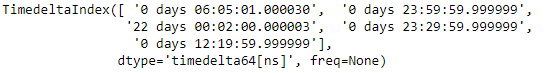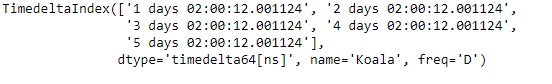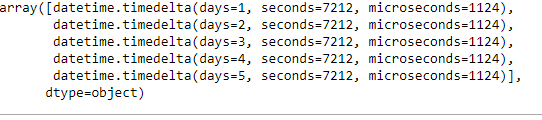Related Articles
Python | Pandas TimedeltaIndex.to_pytimedelta()
• Last Updated : 06 Jan, 2019

Python is a great language for doing data analysis, primarily because of the fantastic ecosystem of data-centric python packages. Pandas is one of those packages and makes importing and analyzing data much easier.

Pandas` TimedeltaIndex.to_pytimedelta()` function return the given TimedeltaIndex object as an ndarray of datetime.timedelta objects.

Syntax : TimedeltaIndex.to_pytimedelta()

Parameters : None

Return : datetimes : ndarray

Example #1: Use `TimedeltaIndex.to_pytimedelta()` function to convert the given TimedeltaIndex object into an ndarray.

 `# importing pandas as pd``import` `pandas as pd`` ` `# Create the TimedeltaIndex object``tidx ``=` `pd.TimedeltaIndex(data ``=``[``'06:05:01.000030'``, ``'+23:59:59.999999'``,``                        ``'22 day 2 min 3us 10ns'``, ``'+23:29:59.999999'``, ``                        ``'+12:19:59.999999'``])`` ` `# Print the TimedeltaIndex object``print``(tidx)`

Output :Now we will use the `TimedeltaIndex.to_pytimedelta()` function to return an ndarray constructed from the given TimedeltaIndex object.

 `# convert to datetime.timedelta``tidx.to_pytimedelta()`

Output :As we can see in the output, the `TimedeltaIndex.to_pytimedelta()` function has returned an array object containing the elements of the given TimedeltaIndex object as object type.

Example #2: Use `TimedeltaIndex.to_pytimedelta()` function to convert the given TimedeltaIndex object into an ndarray.

 `# importing pandas as pd``import` `pandas as pd`` ` `# Create the TimedeltaIndex object``tidx ``=` `pd.TimedeltaIndex(start ``=``'1 days 02:00:12.001124'``, ``                        ``periods ``=` `5``, freq ``=``'D'``, name ``=``'Koala'``)`` ` `# Print the TimedeltaIndex object``print``(tidx)`

Output :Now we will use the `TimedeltaIndex.to_pytimedelta()` function to return an ndarray constructed from the given TimedeltaIndex object.

 `# convert to datetime.timedelta``tidx.to_pytimedelta()`

Output :As we can see in the output, the `TimedeltaIndex.to_pytimedelta()` function has returned an array object containing the elements of the given TimedeltaIndex object as object type.

Attention geek! Strengthen your foundations with the Python Programming Foundation Course and learn the basics.

To begin with, your interview preparations Enhance your Data Structures concepts with the Python DS Course.

My Personal Notes arrow_drop_up